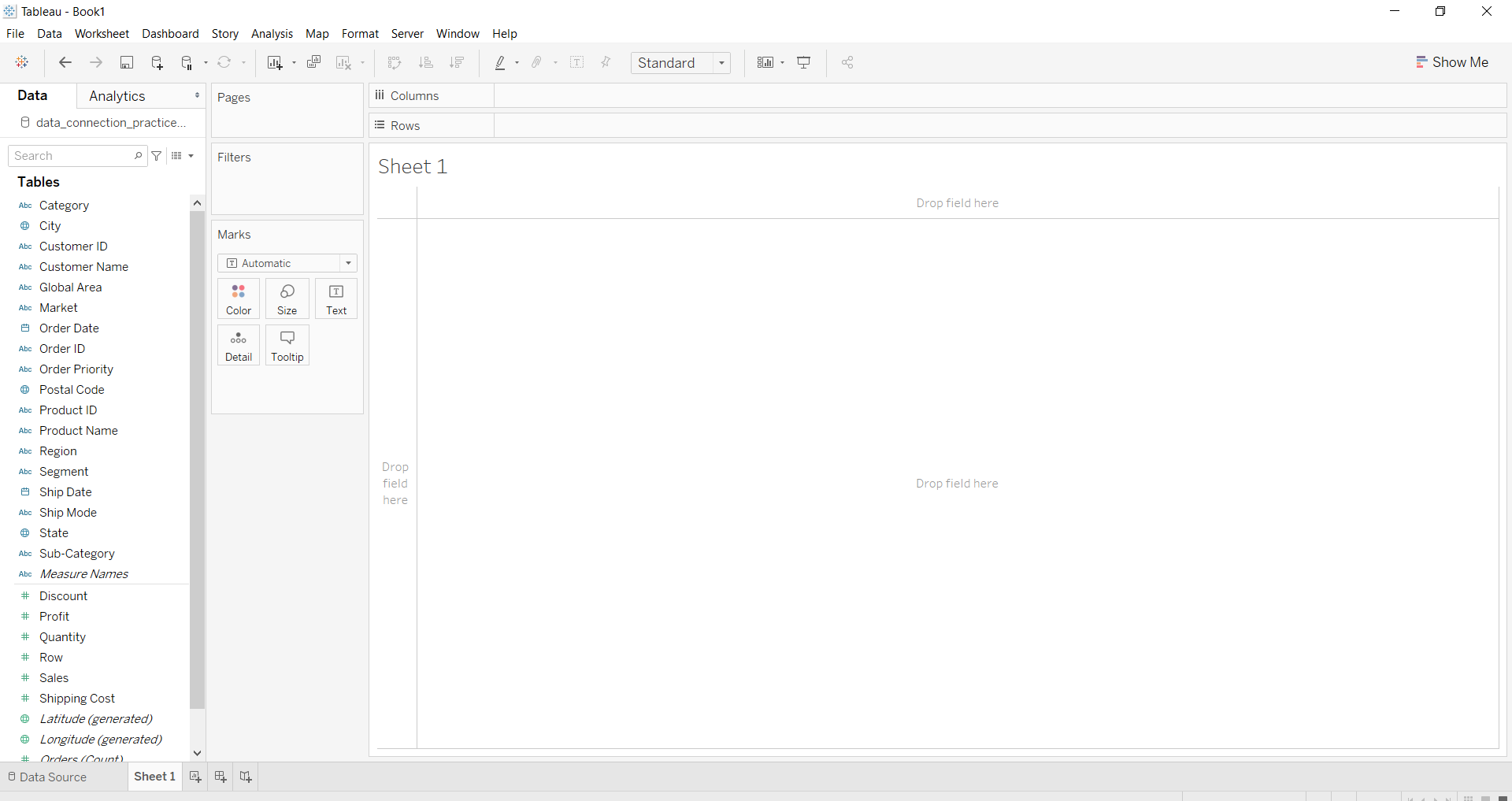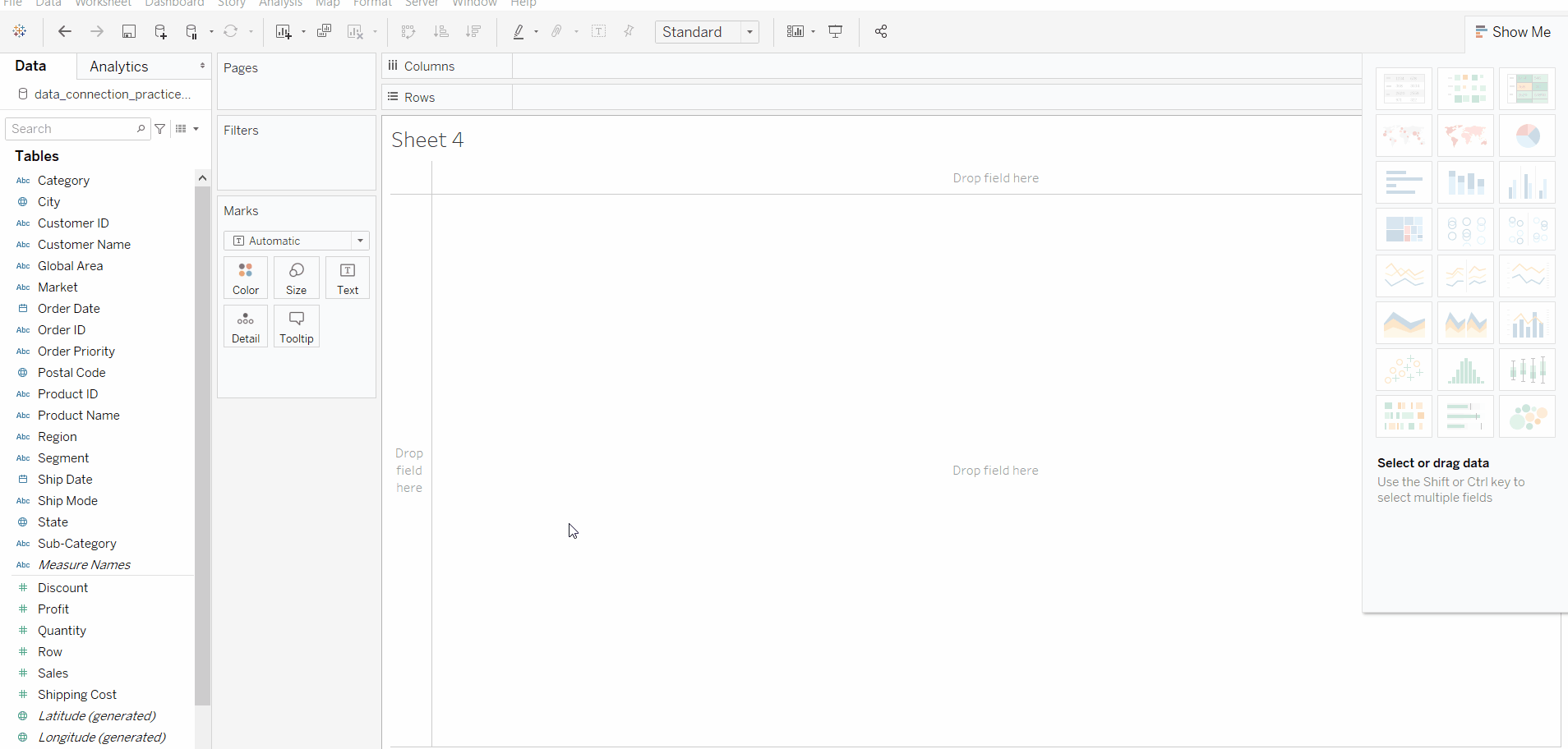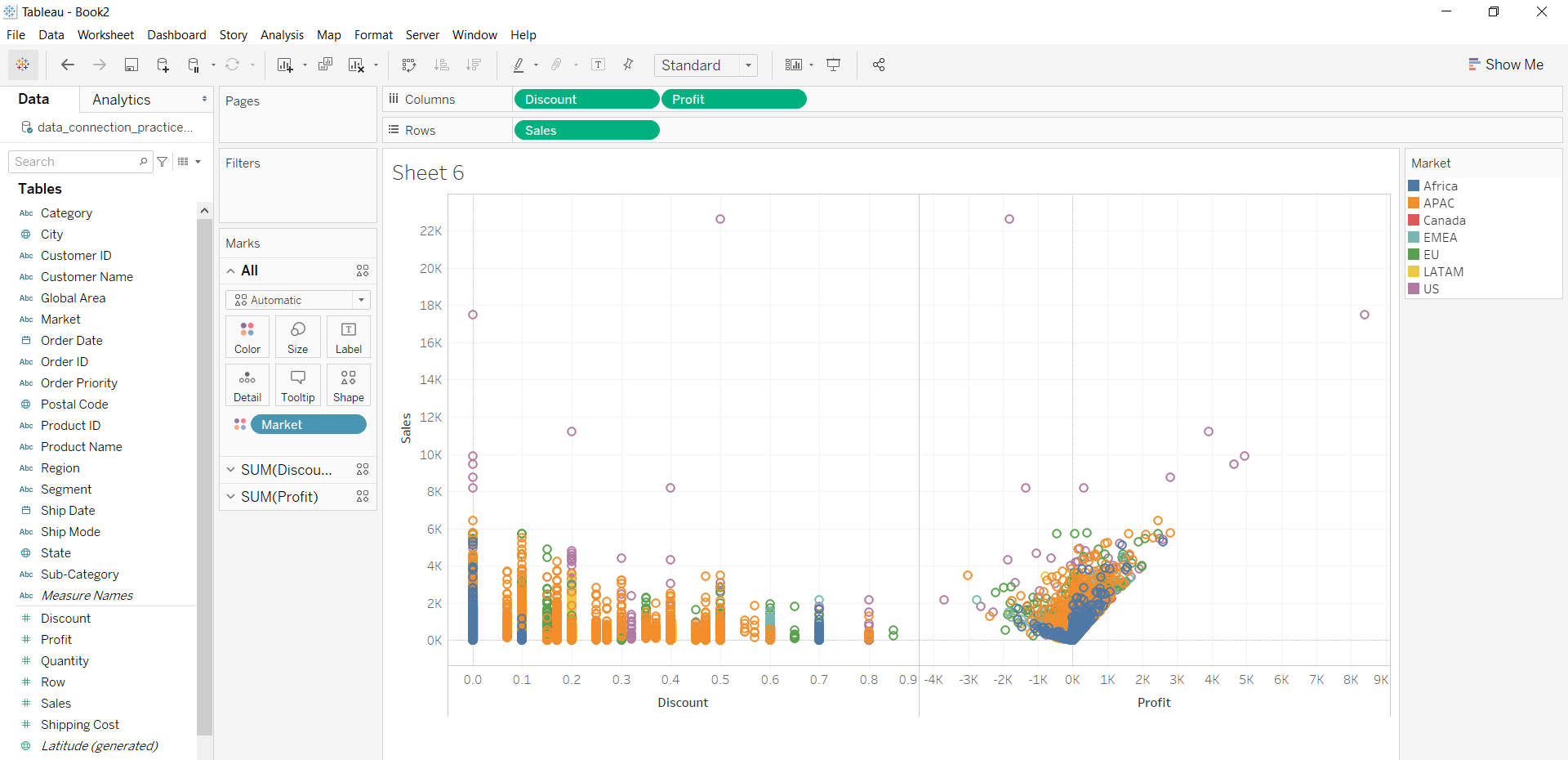# Scatter plot in Tableau

• Last Updated : 01 Oct, 2020

In this article, we will learn how to draw a scatter plot in tableau worksheet to do further operations. For this first look into two terms:

• Tableau: Tableau is a very powerful data visualization tool that can be used by data analysts, scientists, statisticians, etc. to visualize the data and get a clear opinion based on the data analysis. Tableau is very famous as it can take in data and produce the required data visualization output in a very short time.
• Scatter plot: A scatter plot is a set of dotted points to represent individual pieces of data in the horizontal and vertical axis. A graph in which the values of two variables are plotted along the X-axis and Y-axis, the pattern of the resulting points reveals a correlation between them.

Dataset used in the given examples is Dataset.

For this we have to follow some steps :

Open the Tableau tool and connect a dataset into it. Drag and drop the one sheet of the connected dataset. Click on sheet1 to open the tableau worksheet. On clicking Sheet1 you will get whole dataset attributes on the left side and a worksheet for work.To draw a scatter plot, you have to select a minimum of two attributes (one in row and one in column) by drag and drop then select the chart option as scatter plot.

Example 1:Example 2: This example is also drawn similar to above example 1 with some extra marks and which is explained in steps given below :

• Select the Discount, profit with shipping cost and Sales fields as column and row respectively.
• Select the chart type scatter plot.
• Apply marks by color of state.
• Apply marks by details of column values.
• Apply filter of column values.Example 3: This example is also drawn similar to above example 1 with some extra marks and which is explained in steps given below :

• Select the Discount with profit and Sales fields as column and row respectively.
• Select the chart type scatter plot.
• Apply marks by color of market.My Personal Notes arrow_drop_up